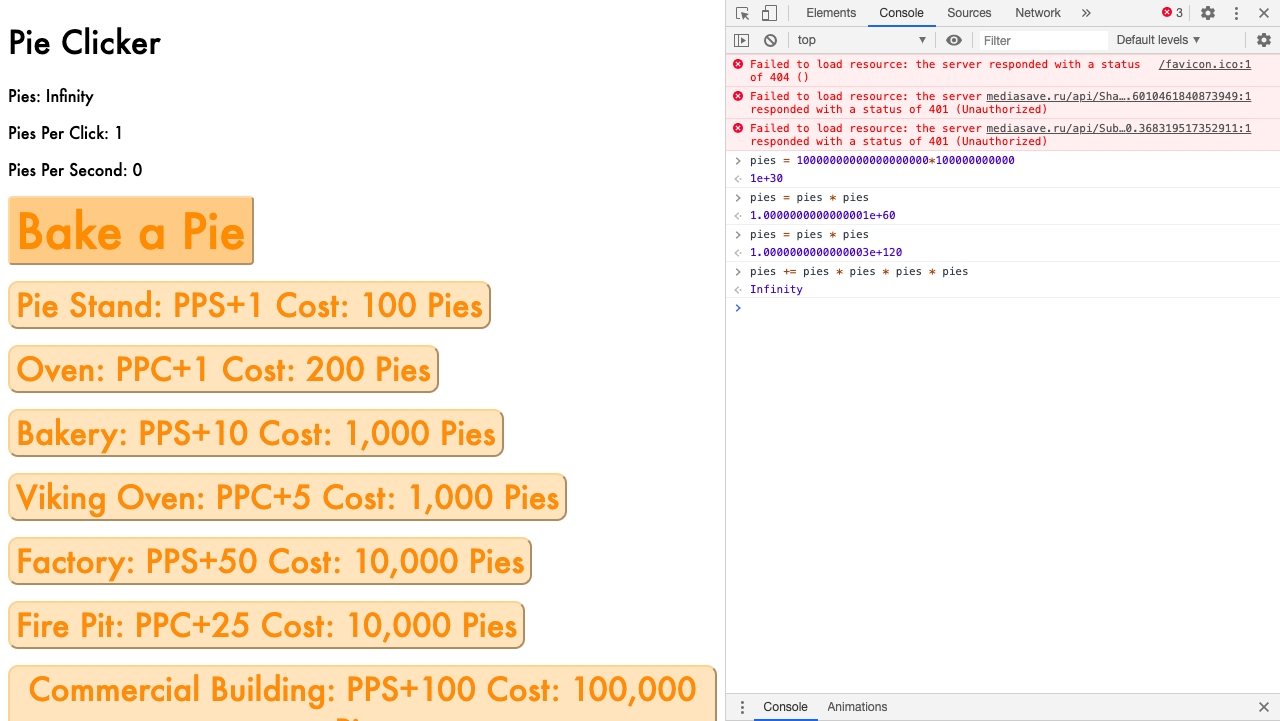The Official Pie Clicker

# My HTML,CSS, JS project #2

The code is ugly I know, but Its my second time making something with CSS, HTML, and JS.

# Please let me know of any ways to make the code look nicer

The code is long and ugly looking, please let me know how I can fix that.

# If you want I will add more buttons

I am open to adding more buttons to make the game last longer

-BobTheTomatoPie

You are viewing a single comment. View All
Viper2211 (56)

# NICE!

By the way, I've hacked it. Here is the code for hacking all of it!

### Click really fast

By the way, it is suggested that you set speed to .01, cause that is the fastest you can go.

``setInterval(b1,<speed>)``

### Get any amount of pies

``pies = <number>``

### All the other buttons for upgrades

Get lots of Pie Stands, while setting their cost to 1 pie

``setInterval(()=>{cos=1;b2();},1)``

Get lots of Ovens, while setting their cost to 1 pie

``setInterval(()=>{cos1=1;b3();},1)``

Get lots of Bakeries, while setting their cost to 1 pie

``setInterval(()=>{cos2=1;b4();},1)``

Get lots of Viking Oven, while setting their cost to 1 pie

``setInterval(()=>{cos3=1;b5();},1)``

Get lots of Factory, while setting their cost to 1 pie

``setInterval(()=>{cos4=1;b6();},1)``

Get lots of Fire Pit, while setting their cost to 1 pie

``setInterval(()=>{cos5=1;b7();},1)``

Get lots of Commercial Building, while setting their cost to 1 pie

``setInterval(()=>{cos6=1;b8();},1)``

Get lots of Pie Labratory, while setting their cost to 1 pie

``setInterval(()=>{cos7=1;b9();},1)``

Get lots of Spaceships, while setting their cost to 1 pie

``setInterval(()=>{cos8=1;b10();},1)``

Get lots of Tomato Pie God, while setting their cost to 1 pie

``setInterval(()=>{cos9=1;b11();},1)``

Note: Setting the price to 1 does not make a difference, because you do not pat for the upgrades.